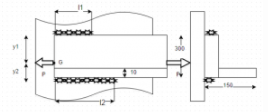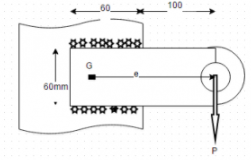Courses

# Test: Unsymmetrically Loaded Welded Joints

## 10 Questions MCQ Test Machine Design | Test: Unsymmetrically Loaded Welded Joints

Description
This mock test of Test: Unsymmetrically Loaded Welded Joints for Mechanical Engineering helps you for every Mechanical Engineering entrance exam. This contains 10 Multiple Choice Questions for Mechanical Engineering Test: Unsymmetrically Loaded Welded Joints (mcq) to study with solutions a complete question bank. The solved questions answers in this Test: Unsymmetrically Loaded Welded Joints quiz give you a good mix of easy questions and tough questions. Mechanical Engineering students definitely take this Test: Unsymmetrically Loaded Welded Joints exercise for a better result in the exam. You can find other Test: Unsymmetrically Loaded Welded Joints extra questions, long questions & short questions for Mechanical Engineering on EduRev as well by searching above.
QUESTION: 1

###The two welds shown in the figure experience equal resisting force?

Solution:

Explanation: As the welded joints are unsymetrically loaded hence resisting forces are different in the two welds.

QUESTION: 2

###The moment of forces about the CG is ?

Solution:

Explanation: External force passes through CG hence moment is zero.

QUESTION: 3

###Which equations can be used to find the resisting force in the two welds?

Solution:

Explanation: One equation is of equilibrium and the other conservation of moment about CG.

QUESTION: 4For the following welded joint l₁y₁=l₂y₂.

Solution:

Explanation: P₁y₁=P₂y₂ and P₁=0.707hl₁τ and P₂=0.707hl₂τ.

QUESTION: 5Find the total length of weld required to with stand the load of 100kN. Permissible shear stress in weld is 70kN/mm².

Solution:

Explanation: 100000=0.707x10xlx70.

QUESTION: 6Find the length of weld 2 required to with stand the load of 100kN. Permissible shear stress in weld is 70kN/mm².

Solution:

Explanation: By finding CG y₂=103.9mm, therefore l₁y₁=l₂y₂ and l₁+l₂=202.06mm.

QUESTION: 7Find the length of weld 1 required to with stand the load of 100kN. Permissible shear stress in weld is 70kN/mm².

Solution:

Explanation: By finding CG y₂=103.9mm, therefore l₁y₁=l₂y₂ and l₁+l₂=202.06mm.

QUESTION: 8The following welded joint is an example of

Solution:

Explanation: The figure clearly depicts.

QUESTION: 9What kind of stresses does the welded joint undergo?

Solution:

Explanation: After shifting force to CG, we have a force and a moment about CG.

QUESTION: 10While considering moment of inertia for calculating torsional shear stress, J=I(xx) + I(yy), which of the following can be neglected in context with the following figure?

Solution:

Explanation: I(xx) is negligible as compared to I(yy) as I(xx)=ltᵌ/12 and t is very less as compared to l so I(xx) is neglected in comparison to I(yy).